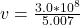Question

Light traveling from water to a gemstone strikes the surface at an angle of 80.0º and has an angle of refraction of 15.2º . (a) What is the speed of light in the gemstone? (b) What is unreasonable about this result? (c) Which assumptions are unreasonable or inconsistent?

1.thongdat2

a

The the speed of light in the gemstone is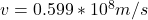b

The unreasonable thing about this is that the speed of ligth in the gemstone is too low

the speed is 19% of the speed of light  which is very low

c

One main unreasonable or inconsistent factor is that the assumption that the  differnce between the angle of incidence and angle of refraction is  very large

Explanation:

From the question we are told that

The  angle of incidence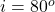The angle of refraction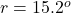From Snell’s law we have ,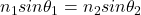Where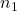is the refractive index of the first medium (water) with a constant value  of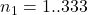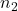is the refractive index of the second medium (gem stone)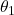is the angle between  the beam and perpendicular surface of the first medium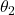is the angle  between the beam an the perpendicular surface of the second medium

Making  thethe subject of the formula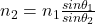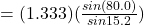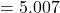Generally refractive index of a material  is mathematically represented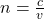Where c is the speed light

v is the speed of light  observed in a medium

Making v the subject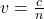substituting value for gem stone